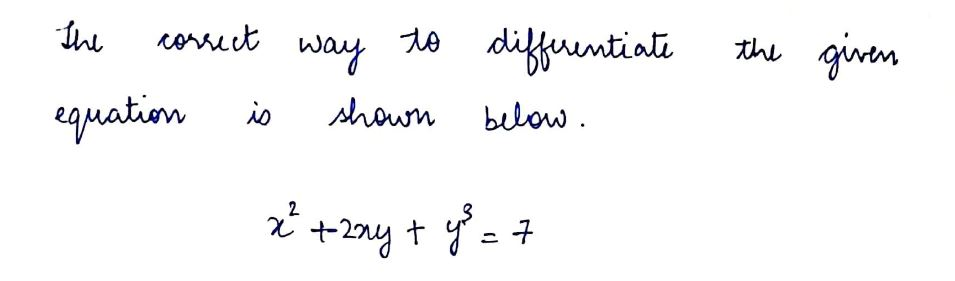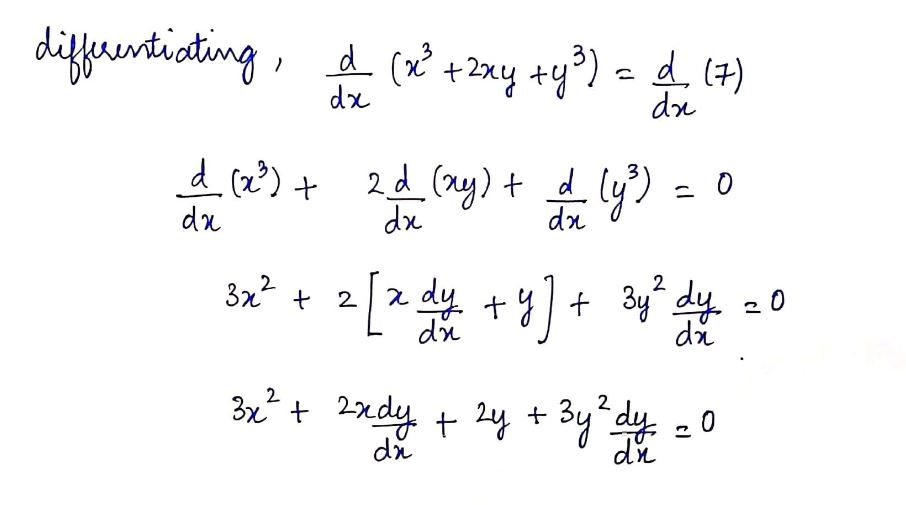# On an exam, Jason was asked to differentiate the equation                                 x2 + 2xy + y3 = 7Find the errors in Jason’s answer: 2x + 2xy , + 3y2 = 0.

Question
1 views

On an exam, Jason was asked to differentiate the equation
x2 + 2xy + y3 = 7
Find the errors in Jason’s answer: 2x + 2xy , + 3y2 = 0.

check_circle

Step 1...

### Want to see the full answer?

See Solution

#### Want to see this answer and more?

Solutions are written by subject experts who are available 24/7. Questions are typically answered within 1 hour.*

See Solution
*Response times may vary by subject and question.
Tagged in